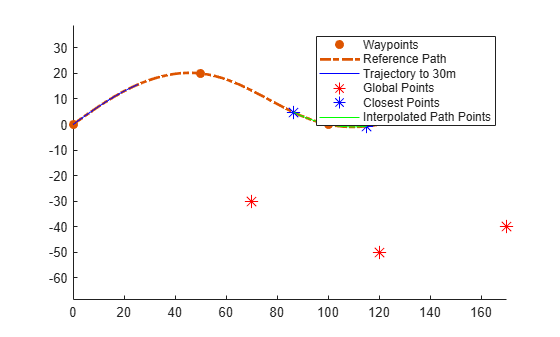# closestPoint

Find closest point on reference path to global point

Since R2020b

## Syntax

``pathPoints = closestPoint(refPath,points)``
``[pathPoints,inWindow] = closestPoint(refPath,points)``
``[_] = closestPoint(refPath,points,searchWindow)``

## Description

example

````pathPoints = closestPoint(refPath,points)` finds the closest point on the reference path to each of the specified (x,y)-positions `points`.```
````[pathPoints,inWindow] = closestPoint(refPath,points)` optionally returns a logical vector `inWindow`, specifying whether each point for the corresponding xy coordinate in `points` is projected within the search window..```
````[_] = closestPoint(refPath,points,searchWindow)` optionally accepts a nondecreasing row vector `searchWindow`, which defines the interval of the path to use to find the closest points.```

## Examples

collapse all

Generate a reference path from a set of waypoints.

```waypoints = [0 0; 50 20; 100 0; 150 10]; refPath = referencePathFrenet(waypoints);```

Create a `trajectoryGeneratorFrenet` object from the reference path.

`connector = trajectoryGeneratorFrenet(refPath);`

Generate a five-second trajectory between the path origin and a point 30 meters down the path as Frenet states.

```initCartState = refPath.SegmentParameters(1,:); initFrenetState = global2frenet(refPath,initCartState); termFrenetState = initFrenetState + [30 zeros(1,5)]; frenetTraj = connect(connector,initFrenetState,termFrenetState,5);```

Convert the trajectory to the global states.

`globalTraj = frenet2global(refPath,frenetTraj.Trajectory);`

Display the reference path and the trajectory.

```show(refPath); axis equal hold on plot(globalTraj(:,1),globalTraj(:,2),'b')```

Specify global points and find the closest points on reference path.

```globalPoints = waypoints(2:end,:) + [20 -50]; nearestPathPoint = closestPoint(refPath,globalPoints);```

Display the global points and the closest points on reference path.

```plot(globalPoints(:,1),globalPoints(:,2),'r*','MarkerSize',10) plot(nearestPathPoint(:,1),nearestPathPoint(:,2),'b*','MarkerSize',10)```

Interpolate between the arc lengths of the first two closest points along the reference path.

```arclengths = linspace(nearestPathPoint(1,6),nearestPathPoint(2,6),10); pathStates = interpolate(refPath,arclengths);```

Display the interpolated path points.

```plot(pathStates(:,1),pathStates(:,2),'g') legend(["Waypoints","Reference Path","Trajectory to 30m",... "Global Points","Closest Points","Interpolated Path Points"])```## Input Arguments

collapse all

Reference path, specified as a `referencePathFrenet` object.

Global points, specified as a P-by-2 numeric matrix with rows of the form `[x y]`. P is the number of points. Positions are in meters.

Search window on path to determine closest points, specified as an two-element row vector.

## Output Arguments

collapse all

Closest points on the reference path , returned as an N-by-6 numeric matrix with rows of form ```[x y theta kappa dkappa s]```, where:

• x y and theta — SE(2) state expressed in global coordinates, with x and y in meters and theta in radians

• kappa — Curvature, or inverse of the radius, in `m-1`

• dkappa — Derivative of curvature with respect to arc length in `m-2`

• s — Arc length, or distance along path from path origin, in meters

N is the number of points sampled along the reference path.

Indication whether each point nearest to the corresponding xy coordinate in `points`, is projected within the search window, returned as an N-element logical column vector, where N is the number of points in `points`. Points being projected within the search window are `true`, or `false` if they lie at the end of a window.

## Version History

Introduced in R2020b